Effective S Chand Class 10 Maths Solutions ICSE Chapter 12 Similar Triangles Ex 12(a) can help bridge the gap between theory and application.

## S Chand Class 10 ICSE Maths Solutions Chapter 12 Similar Triangles Ex 12(a)

Question 1.
Draw rectangular axes. Scale them from 0 to 18. Plot the points:
A (3, 4), B (9, 4), C (9, 6), D (3, 6). Join the points in alphabetical order to form a rectangle. Transform each of the point (x, y) on the points (x1, y1) by the transformation (x, y) → (2x, 2y)
Lable image A1B1C1D1 to correspond with ABCD.
(i) What do you notice about the length of each side of the image when you compare it with the corresponding side of the original rectangle?
(ii) Are the rectangles similar in shape?
(iii) Write the ratio $$\frac{\text { Area of image } A_1 B_1 C_1 D_1}{\text { Area of original } A B C D}$$ in the lowest terms.
Solution:Plot the points A (3, 4), B (9, 4), C (9, 6), D (3, 6) on the graph as shown
Join them to form rectangle ABCD
The ratio scale of transformation is (x, y) → (2x, 2y) i.e. 1 : 2
Now plot the points A’ (6, 8), B’ (18, 8), C’ (18, 12) and D’ (6, 12) on the same graph and join them to form another rectangle A’B’C’D’

• Length of the sides of the image of rectangle ABCD is 1 : 2 i.e. double of the original rectangle
• Yes the rectangles are similar and ratio is 1 : 2
• Ratio in area = $$\frac{\text { Area of image } A_1 B_1 C_1 D_1}{\text { Area of original } A B C D}=\frac{12 \times 4}{6 \times 2}=\frac{48}{12}=\frac{4}{1}$$
i.e. 4 : 1

Question 2.
(a) What would be the effect (i) on the length of sides, (ii) on the area of the transformation (x, y) → (3x, 3y) ?
Label the image A1B1C1D1 to correspond with ABCD.
(b) Which transformation would make the area of the image sixteen times the area of original rectangle?
(c) Which transformation would made the length of each side of the image half the length of the corresponding side of the original rectangle ?
Solution:
(i) ∵ (x, y) → (3x, 3y)
∴ The image A1B1C1D1 is three times in length of the figure ABCD

(ii) ∵ The area of the image is 16 times the area of the original rectangle
∴ (x, y) → (4x, 4y) (∵ $$\sqrt{16}$$ = 4)

(iii) In the given figure,
The image is half the length of the corres-ponding sides of the original figure
∴ (x, y) → ($$\frac { 1 }{ 2 }$$x, $$\frac { 1 }{ 2 }$$y)

Question 3.
In the figure, O is the centre of dilatation and the dilatation factor is 3.
(i) Complete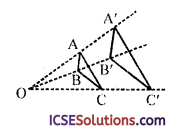Preimage Image 1. AB = 5 units A’B’ = ? 2. BC = ? B’C’ = 18 units 3. AC = 2 units A’C’ = ? 4. OA = 7 units OA’ = ? 5. OC = ? OC’ = 39 units 6. OB = m units m ∈ R OB’ = ?

(ii) Determine:
(a) $$\frac{\mathrm{OA}^{\prime}}{\mathrm{OA}}$$
(b) $$\frac{\mathrm{OB}^{\prime}}{\mathrm{OB}}$$
(c) $$\frac{\mathrm{OC}^{\prime}}{\mathrm{OC}}$$
(d) $$\frac{\mathrm{A}^{\prime} \mathrm{B}^{\prime}}{\mathrm{AB}}$$
(e) $$\frac{\mathrm{B}^{\prime} \mathrm{C}^{\prime}}{\mathrm{BC}}$$
(f) $$\frac{C^{\prime} A^{\prime}}{C A}$$
Solution:
O is the centre of dilatation and the dilatation factor is 3
i.e. (x, y) → (3x, 3y)

 Preimage Image 1. AB = 5 units A’B’ = 3 x 5 = 15 units 2. BC = $$\frac { 1 }{ 3 }$$ x 18 = 6 units B’C’ = 18 units 3. AC = 2 units A’C’ = 3 x 2 = 6 units 4. OA = 7 units OA’ = 3 x 7 = 21 units 5. OC = $$\frac { 1 }{ 3 }$$ x 39 = 13 units OC’ = 39 units 6. OB = m units m ∈ R OB’ = m x 3 = 3 m units m ∈ R

(ii) ∵ (x, y) → (3x, 3y)
(a) $$\frac{\mathrm{OA}^{\prime}}{\mathrm{OA}}$$ = $$\frac { 3 }{ 1 }$$
(b) $$\frac{\mathrm{OB}^{\prime}}{\mathrm{OB}}$$ = $$\frac { 3 }{ 1 }$$
(c) $$\frac{\mathrm{OC}^{\prime}}{\mathrm{OC}}$$ = $$\frac { 3 }{ 1 }$$
(d) $$\frac{\mathrm{A}^{\prime} \mathrm{B}^{\prime}}{\mathrm{AB}}$$ = $$\frac { 3 }{ 1 }$$
(e) $$\frac{\mathrm{B}^{\prime} \mathrm{C}^{\prime}}{\mathrm{BC}}$$ = $$\frac { 3 }{ 1 }$$
(f) $$\frac{C^{\prime} A^{\prime}}{C A}$$ = $$\frac { 3 }{ 1 }$$

Question 4.
A triangle ABC has its vertices at (1, 1), (1, 3) and (3, 1) respectively. Enlarge this triangle by using a scale factor of 2 with the origin as the centre of enlargement.
Solution:
The coordinates of the vertices of a triangle are A (1, 1), B (1, 3) and C (3, 1) respectively
Enlargement of scale factor is 2 i.e. (x, y) → (2x, 2y)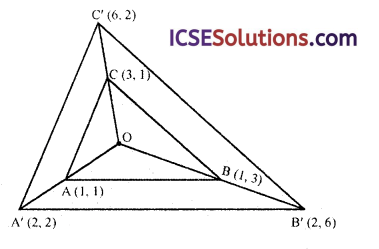∴ Coordinates of enlargement triangle will be
A’ (2, 2), B’ (2, 6) and C’ (6, 2) respectively. O is the origin (centre) of the enlargement triangle whose vertices are A’ (2, 2), B’ (2, 6) and C’ (6, 2)

Question 5.
The coordinates of vertices being given, enlarge the given figure by using the given scale factor. For each question you may use a grid.

 Coordinates of vertices Scale factor Scale factor (a) P (1, 2), Q (2, 2), R (2, 1) 4 4 (b) A (0, 0), B (3, 2), C (4, 0) 2 2 (c) A (5, 5), B (5, 7), C (7, 7), D (7, 5) 4 4 (d) A (5, 4) X (5, 8), Y (7, 8), Z (7, 4) 2 2

Solution:
(a) Coordinates of vertices are P (1, 2), Q (2, 2), R (2, 1)
Scale factor 1 : 4
and centre of enlargement is origin (0, 0)
∴ (x, y) → (4x, 4y)
∴ Coordinates of the enlarged figure will be P’ (4, 8), Q’ (8, 8) and R’ (8, 4)

(b) Coordinates of vertices are A (0, 0), B (3, 2), C (4, 0)
Scale factor is 1 : 2
(x, y) → (2x, 2y)
∴ Coordinates of enlarged figure will be A’ (0, 0), B’ (6, 4) and C’ (8, 0)

(c) Coordinates of figures are A (5, 5), B (5, 7), C (7, 7) and D (7, 5)
and enlargement factor is 1 : 4
∴ (x, y) → (4x, 4y)
and centre of enlargement is the point (6, 6)
∴ Co-ordinates of the vertices of enlarged figure will be A’ (2,2), B’ (10, 2), C’ (10, 10) and D’ (2,10)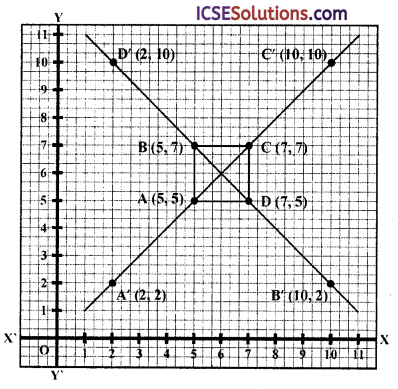(d) Coordinates of figure are A (5, 4), X (5, 8), Y (7, 8) and Z (7, 4)
and enlargement factor is 1 : 2 and centre of enlargement is the point (6, 6)
The vertices of the enlarged figure A’X’Y’Z’ will be A’ (4, 2), X’ (4, 10), Y’ (8, 10) and Z’ (8, 2)Question 6.
Draw the image of the shape KLMN after an enlargement by scale factor $$\frac { 1 }{ 2 }$$ with centre C. Label the image K’L’M’N’.
Solution:
Take CK = 6 cm and take its mid point K’ such that CK’ = 3 cm.
Join CK, LM, MC and NC.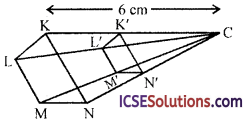Draw K’L’ || KL, K’N’ || KN, N’M’ || NM and L’M’ || LM.
Then figure, K’L’M’N’ is the image of figure KLMN by the scale factor $$\frac { 1 }{ 2 }$$ with centre C.

Question 7.
Figure shows the enlargement transformation of shape ABCD to PQRS by a scale factor of 2. Find the centre of enlargement and state its coordinates.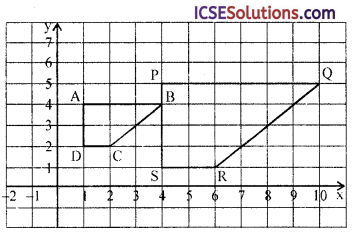Solution:
Join PA, QB, RC and SD and produce them. Which meet all of them at a point L. This is the required centre of enlargement and its co-ordiantes are (-2, 3).Question 8.
A square with side 3 cm is drawn any where in a plane which is then enlarged about any point in the plane with a scale factor 2. What is the area of the image of the square?
Solution:
Side of a square = 3 cm
When it is enlarged, the scale factor = 2
i.e. k = 2
Area of the square = (side)² = (3)² = 9 cm²
∴ Area of the image of square = k² (Area of square)
= (2)² x 9 = 4 x 9 = 36 cm²

Question 9.
Construct a square ABCD of side 2 cm. Enlarge ABCD with enlargement factor m = 2 and label its image A’B’C’D’. What is the relation between the area ABCD and its image A’B’C’D’.
Solution:
Draw a square ABCD with each side 2 cm. Join AC and BD and produced them both sides. .
Take A’B’ = 4 cm (as m = 2) and complete the required square A’B’C’D’ with each side 4 cm.
Now area of square ABCD = 2 x 2 = 4 cm²
and area of square A’B’C’D’ with each side 2 x 2 = 4 cm
= 4 x 4 = 16 cm²
Hence, area of the image of square ABCD = 16 cm²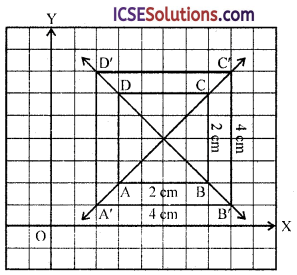Question 10.
A triangle, whose area is 12 cm², is transformed under enlargement about a point in space. If the area of its image is 108 cm², find the scale factor of the enlargement.
Solution:
Area of the triangle = 12 cm²
Area of enlarged triangle = 108 cm² .
Let scale factor = k and then scale factor of area = k²
Then k² x 12 = 108 ⇒ k² = $$\frac { 108 }{ 12 }$$ = 9
∴ k = $$\sqrt{9}$$ = 3

Question 11.
The parallelogram ABCD has vertices (6, 3), (9, 3), (12, 9), (9, 9) respectively. Copy the grid and the parallelogram. An enlargement scale factor $$\frac { 1 }{ 3 }$$ and centre (0, 0) transforms parallelogram ABCD onto parallelogram A’B’C’D’.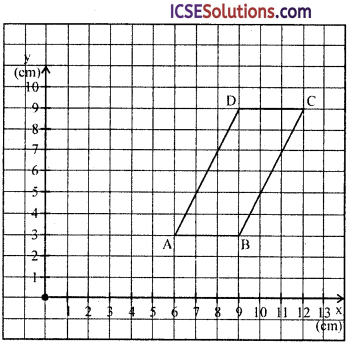(a) (i) Draw the parallelogram A’B’C’D’.
(ii) Calculate the area of parallelogram A’B’C’D’.

(b) The side AB has length 3 cm. The original shape ABCD is now enlarged with a scale factor of $$\frac { 2 }{ 5 }$$ to give A”B”C”D”. Calculate the length of the side A”B”.
Solution:
A parallelogram ABCD is given whose vertices are A (6, 3), B (9, 3), C (12, 3), D (9, 9) scale factor is $$\frac { 1 }{ 3 }$$ and centre O (0, 0) transforms parallelogram ABCD on to ||gm A’B’C’D’.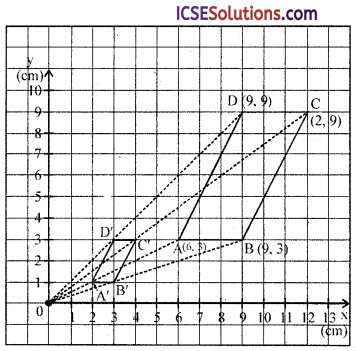(a) (i) Join OA, OB, OC and OD.
Take a point A’ on OA such that OA’ = $$\frac { 1 }{ 3 }$$ OA
Similarly take points B’, C’ and D’
Join A’B’, B’C’, C’D’ and D’A’
A’B’C’D’ is the required || gm

(ii) Area of ||gm ABCD = A’B’ x Perpendicular between A’B’ and C’D’ =1 x 2 = 2 cm²

(b) Let AB = 3 cm
Now ||gm ABCD is enlarged with a scale factor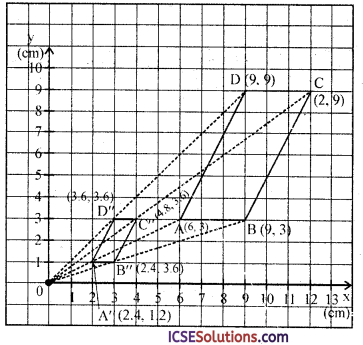$$\frac { 2 }{ 5 }$$ given new ||gm A”B”C”D”.
Length of side A”B” = 1.2 cm
Join OA, OB, OC and OD
Take a point A” (6 x $$\frac { 2 }{ 5 }$$, 3 x $$\frac { 2 }{ 5 }$$) or (2.4, 1.2)
Similarly point B” (2.4, 1.2), C” (3.6, 3.6) and D” (4.8,3.6) and join A”B”, B”C”, C”D”, D”A” respectively.
A”B”C”D” is the required parallelogram.
On measuring length of A”B” = 1.2 cm.

Question 12.
A model of a van is constructed using a scale of 1 : 40. If the length of the real van is 8 metres, what is the length of the model?
Solution:
Scale in model of a van is the real van = 1 : 40
Length of the real van = 8 m
∴ Length of the model = $$\frac { 8×1 }{ 40 }$$ m
= $$\frac { 8×100 }{ 40 }$$ = 20cm

Question 13.
Akhil is drawing a plan of his classroom using a scale of 1 : 50. If the length and width of the classroom are 8 metres and 6 metres, how long will they each be on his drawing?
Solution:
Scale of the plan of a classroom = 1 : 50
Length of classroom = 8 m
∴ Length of the drawing of the room = $$\frac { 8×1 }{ 50 }$$ x 100 cm = 16 cm
and breadth = $$\frac { 6×1 }{ 50 }$$ x 100 = 12 cm

Question 14.
Nikhil is drawing a plan of the gymnasium at his school using a scale of 1 : 75. If the dimensions of the gymnasium on his plan are 28 cm and 16 cm, what are the dimensions of the real gymnasium?
Solution:
Scale of the plan of the gymnasium of the school = 1 : 75
In the plan,
Length = 28 cm and breadth = 16 cm
∴ Length of actual gymnasium = $$\frac { 28×75 }{ 1 }$$ cm
= $$\frac { 28×75 }{ 100 }$$ m = 21 m
and breadth = $$\frac { 16×75 }{ 100 }$$ = 12 m
∴ Dimension of gymnasium = 21 m by 12 m

Question 15.
Krishna has a model of the Eiffel Tower which is 16 cm high. If the height of the real tower is 320 m, what is the scale of the model?
Solution:
Model of a Eiffel Tower = 16 cm high
But real height = 320 m
∴ Scale = 16 : 320 x 100
= 1 : 20 x 100
= 1 : 2000

Question 16.
The dimensions of the model of a multi-storey building are 1.2 m x 75 cm x 2 m. If the scale factor is 1 : 30, find the actual dimensions of the building.
Solution:
Dimension of the model of a multi-storey.
building are 1.2 m x 75 cm x 2 m
= 120 cm x 75 cm x 200 cm
Scale = 1 : 30, then
Actual length = $$\frac { 12×30 }{ 1 }$$ cm
= $$\frac { 12×30 }{ 100 }$$ = 36 m
Breadth = $$\frac { 375×30 }{ 100 }$$ = $$\frac { 45 }{ 2 }$$ = 22.5 m
Height = $$\frac { 20×30 }{ 100 }$$ = 60 m
Hence, dimensions are 36 m x 22.5 m x 60 m

Question 17.
On a map drawn to a scale of 1 : 2500, a triangular plot of land has the following measurements: AB = 3 cm, BC = 4 cm and ∠ABC = 90°.
(i) Calculate the actual lengths of AB and AC in km;
(ii) The actual area of the plot in km².
Solution:
Scale of map = 1 : 2500
Dimension of a triangular plot are AB = 3 cm, BC = 4 cm and ∠ABC = 90°(i) ∴ Actual length of AB
= $$\frac{3 \times 2500}{1}$$cm
= $$\frac{3 \times 2500}{100}$$ = 75 m
and length of BC = $$\frac{4 \times 2500}{100}$$ = 100 m

(ii) Actual area = $$\frac { 1 }{ 2 }$$ x BC x AB
= $$\frac { 1 }{ 2 }$$ x 75 x 100 m²
= 3750 m²
= $$\frac{3750}{1000 \times 1000}$$ = 0003750 km²
= 0003750 km²

Question 18.
The scale of a map is 1 : 200000. A plot of land of area 20 km² is to be represented on the map; find :
(i) the number of kilometres on the ground which is represented by 1 centimetre on the map;
(ii) the area in km² that can be represented by 1 cm²;
(iii) the area on the map that represents the plot of land.
Solution:
It means that 1 cm on the map will represent 200000 cm 2 on the ground
(i) ∴ 1 cm = 20000 cm
= $$\frac{200000}{100 \times 1000}$$ km = 2 km

(ii) ∵ 1 cm on the map = 2 km on the ground and 1 cm² = 2 x 2 = 4 km² on the ground

(iii) Area of a plot on the ground = 20 km²
Area of plot to be represented on the map will be = $$\frac { 20 }{ 4 }$$ = 5 cm²

Question 19.
A model of a ship is made to a scale of 1 : 200.
(i) The length of the model is 4 m. Calculate the length of the ship.
(ii) The area of the deck of the ship is 160000 m². Find the area of the deck of the-model.
(iii) The volume of the model is 200 litres. Calculate the volume of the ship in m³.
Solution:
Scale of the model of a ship = 1 : 200
(i) Length of model = 4 m
∴ Actual length = $$\frac { 4×200 }{ 1 }$$ = 800 m

(ii) Area of deck = 160000 m2
∴ Area on the model = $$\frac { 160000 }{ 200× }$$ = 4 m²

(iii) Volume of model = 200 litres
= $$\frac { 200 }{ 1000 }$$ = 0.2 m²
∴ Volume of actual ship = 1 m³ = 1000 l = 0.2 x (200)³ m³
= $$\frac { 2 }{ 10 }$$ x 200 x 200 x 200 = 1600000 m³